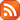Subscribe by RSS

# Department Colloquium

Fri., Mar. 5, 2021 3:30 p.m.

Location: Live Stream

Speaker: Seyed Ahmad Mojallal, University of Regina

Title:  Applications of the vertex-clique incidence matrix of a graph (487 kB)Abstract:

In this talk, we make use of an interaction between the theory of clique partitions of a graph and graph spectra. We use the theory of clique partitions and introduce the notion of a vertex-clique incidence matrix of the graph. We give new lower bounds for the negative eigenvalues and negative inertia of a graph. Moreover, utilizing vertex-clique incidence matrices, we generalize several notions such as the signless Laplacian matrix and a line graph of a graph as well as the incidence energy and the signless Laplacian energy of the graph.

Applying a similar type of incidence matrices obtained from the theory of clique covering, we report on some recent research studying the minimum number of distinct eigenvalues of a graph.

This is joint work with Shaun Fallat.